• Matlab余弦相似度算法判断图片相似度并识别源代码 Matlab 余弦相似度 图像匹配 可直接运行 Matlab余弦相似度算法判断图片相似度并识别源代码 Matlab 余弦相似度 图像匹配 可直接运行
• 余弦相似度算法: 余弦相似性通过测量两个向量的夹角的余弦值来度量它们之间的相似性。0度角的余弦值是1，而其他任何角度的余弦值都不大于1；并且其最小值是-1。从而两个向量之间的角度的余弦值确定两个向量是否...
余弦相似度算法:
余弦相似性通过测量两个向量的夹角的余弦值来度量它们之间的相似性。0度角的余弦值是1，而其他任何角度的余弦值都不大于1；并且其最小值是-1。从而两个向量之间的角度的余弦值确定两个向量是否大致指向相同的方向。两个向量有相同的指向时，余弦相似度的值为1；两个向量夹角为90°时，余弦相似度的值为0；两个向量指向完全相反的方向时，余弦相似度的值为-1。这结果是与向量的长度无关的，仅仅与向量的指向方向相关。余弦相似度通常用于正空间，因此给出的值为-1到1之间。
坐标系表示：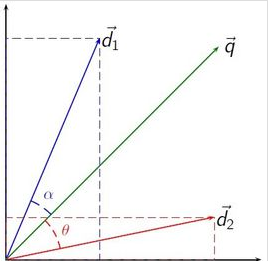具体公式: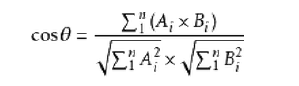具体实现(Java)：
import java.util.ArrayList;

public class SimilarityUtil {

public static double similarity(ArrayList va, ArrayList vb) {
if (va.size() > vb.size()) {
int temp = va.size() - vb.size();
for (int i = 0; i < temp; i++) {
}
} else if (va.size() < vb.size()) {
int temp = vb.size() - va.size();
for (int i = 0; i < temp; i++) {
}
}

int size = va.size();
double simVal = 0;

double num = 0;
double den = 1;
double powa_sum = 0;
double powb_sum = 0;
for (int i = 0; i < size; i++) {
double a = Double.parseDouble(va.get(i).toString());
double b = Double.parseDouble(vb.get(i).toString());

num = num + a * b;
powa_sum = powa_sum + (double) Math.pow(a, 2);
powb_sum = powb_sum + (double) Math.pow(b, 2);
}
double sqrta = (double) Math.sqrt(powa_sum);
double sqrtb = (double) Math.sqrt(powb_sum);
den = sqrta * sqrtb;

simVal = num / den;

return simVal;
}
}

案例分析:
姓名/兴趣  吃苹果  逛商店  看电视剧  打羽毛球  吃桔子  小红  3.5  5  5  5  0                    xxx  3.5  5  5  0  0
分别以小红和xxx的兴趣评分组成向量va和vb，运用多维余弦相似公式，设向量 A =
(A1,A2,...,An)，B = (B1,B2,...,Bn) ：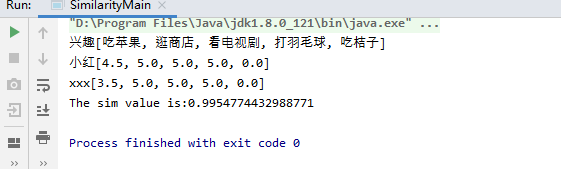计算得相似度为:0.9954774432988771
具体实现代码：
import java.util.ArrayList;

public class SimilarityMain {

public static double similarity(ArrayList va, ArrayList vb) {
if (va.size() > vb.size()) {
int temp = va.size() - vb.size();
for (int i = 0; i < temp; i++) {
}
} else if (va.size() < vb.size()) {
int temp = vb.size() - va.size();
for (int i = 0; i < temp; i++) {
}
}

int size = va.size();
double simVal = 0;

double num = 0;
double den = 1;
double powa_sum = 0;
double powb_sum = 0;
for (int i = 0; i < size; i++) {
double a = Double.parseDouble(va.get(i).toString());
double b = Double.parseDouble(vb.get(i).toString());

num = num + a * b;
powa_sum = powa_sum + (double) Math.pow(a, 2);
powb_sum = powb_sum + (double) Math.pow(b, 2);
}
double sqrta = (double) Math.sqrt(powa_sum);
double sqrtb = (double) Math.sqrt(powb_sum);
den = sqrta * sqrtb;

simVal = num / den;

return simVal;
}

public static void main(String[] args) {
String item[] = {"吃苹果", "逛商店", "看电视剧", "打羽毛球", "吃桔子"};
float a[] = {(float) 4.5, 5, 5, 5,0};
float b[] = {(float) 3.5, 5, 5, 5,0};
ArrayList vitem = new ArrayList();
ArrayList<Float> va = new ArrayList();
ArrayList<Float> vb = new ArrayList();
for (int i = 0; i < a.length; i++)
{
}
System.out.print("兴趣");
System.out.println(vitem);
System.out.print("小红");
System.out.println(va);
System.out.print("xxx");
System.out.println(vb);

SimilarityMain sim = new SimilarityMain();

double simVal = sim.similarity(va, vb);

System.out.println("The sim value is:" + simVal);
}

}

源码gitee地址:
https://gitee.com/jockhome/
展开全文• 使用余弦相似度算法计算文本相似度 </h1> <div class="clear"></div> <div class="postBody"> 在工作中一直使用余弦相似度算法计算两段文本的相似度和两个用户的相似度。一直弄不明白...


使用余弦相似度算法计算文本相似度
        </h1>
<div class="clear"></div>
<div class="postBody">


在工作中一直使用余弦相似度算法计算两段文本的相似度和两个用户的相似度。一直弄不明白多维的余弦相似度公式是怎么推导来的。今天终于花费时间把公式推导出来，其实很简单，都是高中学过的知识，只是很多年没用了，都还给老师了。本文还通过一个例子演示如果使用余弦相似度计算两段文本的相似度。

余弦函数在三角形中的计算公式为：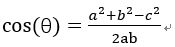在直角坐标系中，向量表示的三角形的余弦函数是怎么样的呢？下图中向量a用坐标(x1,y1)表示，向量b用坐标(x2,y2)表示。

向量a和向量b在直角坐标中的长度为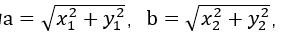向量a和向量b之间的距离我们用向量c表示，就是上图中的黄色直线，那么向量c在直角坐标系中的长度为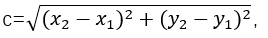，将a，b，c带入三角函数的公式中得到如下的公式：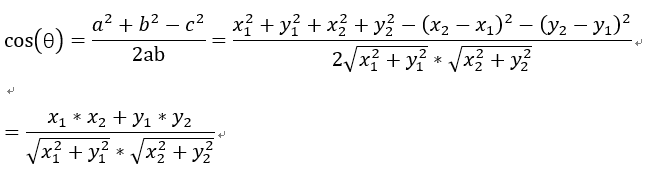这是2维空间中余弦函数的公式，那么多维空间余弦函数的公式就是：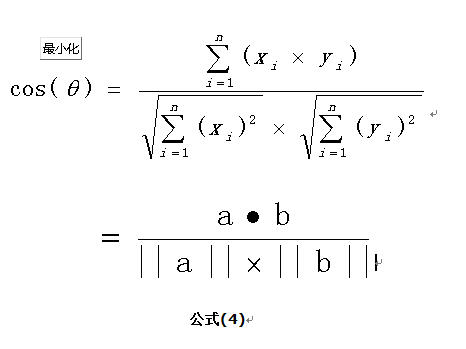余弦相似度

余弦相似度量：计算个体间的相似度。

相似度越小，距离越大。相似度越大，距离越小。

假设有3个物品，item1，item2和item3，用向量表示分别为：

item1[1,1,0,0,1]，

item2[0,0,1,2,1]，

item3[0,0,1,2,0]，

即五维空间中的3个点。用欧式距离公式计算item1、itme2之间的距离，以及item2和item3之间的距离，分别是：

item1-item2=item2-item3=用余弦函数计算item1和item2夹角间的余弦值为：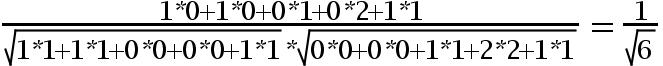用余弦函数计算item2和item3夹角间的余弦值为：由此可得出item1和item2相似度小，两个之间的距离大(距离为7)，item2和itme3相似度大，两者之间的距离小(距离为1)。

余弦相似度算法：一个向量空间中两个向量夹角间的余弦值作为衡量两个个体之间差异的大小，余弦值接近1，夹角趋于0，表明两个向量越相似，余弦值接近于0，夹角趋于90度，表明两个向量越不相似。

余弦相似度

余弦相似度量：计算个体间的相似度。

相似度越小，距离越大。相似度越大，距离越小。

余弦相似度算法：一个向量空间中两个向量夹角间的余弦值作为衡量两个个体之间差异的大小，余弦值接近1，夹角趋于0，表明两个向量越相似，余弦值接近于0，夹角趋于90度，表明两个向量越不相似。

下面我们介绍使用余弦相似度计算两段文本的相似度。思路：1、分词；2、列出所有词；3、分词编码；4、词频向量化；5、套用余弦函数计量两个句子的相似度。

句子A：这只皮靴号码大了。那只号码合适。

句子B：这只皮靴号码不小，那只更合适。

1、分词：

使用结巴分词对上面两个句子分词后，分别得到两个列表：

listA=[‘这‘, ‘只‘, ‘皮靴‘, ‘号码‘, ‘大‘, ‘了‘, ‘那‘, ‘只‘, ‘号码‘, ‘合适‘]

listB=[‘这‘, ‘只‘, ‘皮靴‘, ‘号码‘, ‘不小‘, ‘那‘, ‘只‘, ‘更合‘, ‘合适‘]

2、列出所有词，将listA和listB放在一个set中，得到：

set={'不小', '了', '合适', '那', '只', '皮靴', '更合', '号码', '这', '大'}

将上述set转换为dict，key为set中的词，value为set中词出现的位置，即‘这’:1这样的形式。

dict1={'不小': 0, '了': 1, '合适': 2, '那': 3, '只': 4, '皮靴': 5, '更合': 6, '号码': 7, '这': 8, '大': 9}，可以看出“不小”这个词在set中排第1，下标为0。

3、将listA和listB进行编码，将每个字转换为出现在set中的位置，转换后为：

listAcode=[8, 4, 5, 7, 9, 1, 3, 4, 7, 2]

listBcode=[8, 4, 5, 7, 0, 3, 4, 6, 2]

我们来分析listAcode，结合dict1，可以看到8对应的字是“这”，4对应的字是“只”，9对应的字是“大”，就是句子A和句子B转换为用数字来表示。

4、对listAcode和listBcode进行oneHot编码，就是计算每个分词出现的次数。oneHot编号后得到的结果如下：

listAcodeOneHot = [0, 1, 1, 1, 2, 1, 0, 2, 1, 1]

listBcodeOneHot = [1, 0, 1, 1, 2, 1, 1, 1, 1, 0]

下图总结了句子从分词，列出所有词，对分词进行编码，计算词频的过程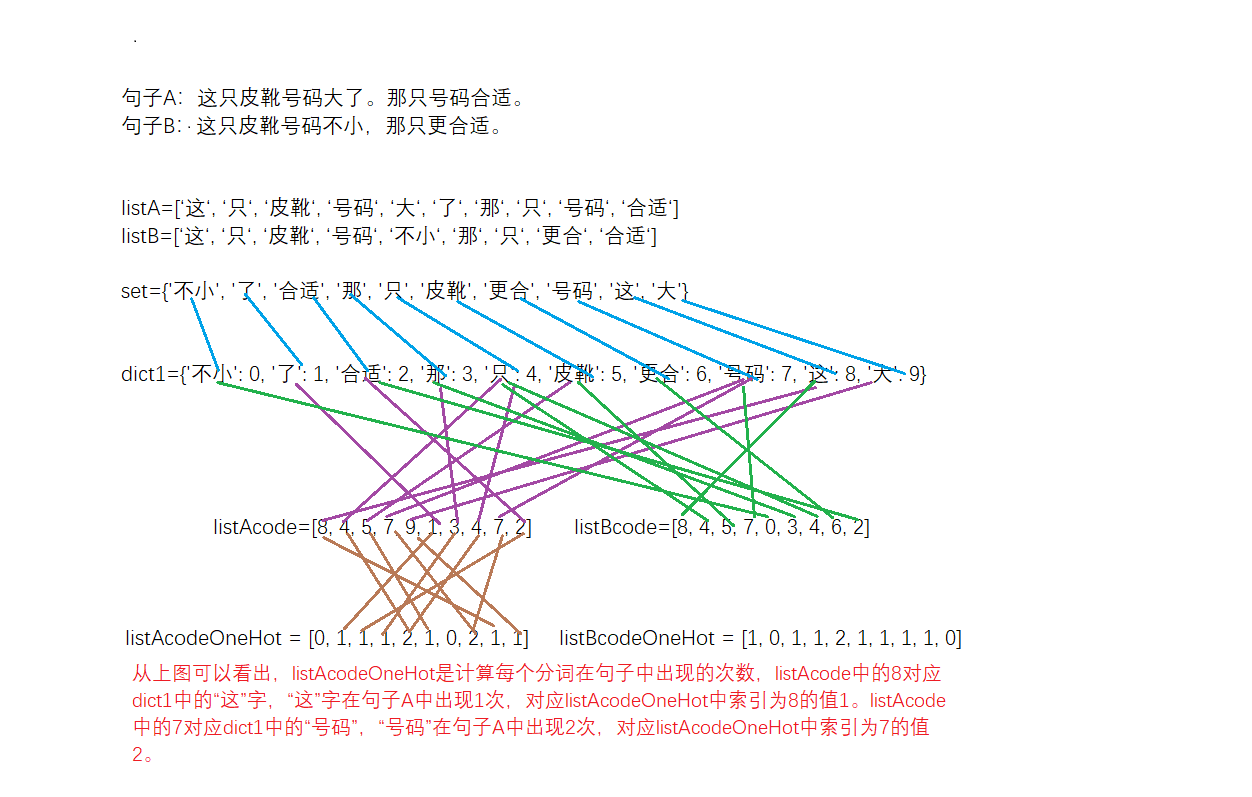5、得出两个句子的词频向量之后，就变成了计算两个向量之间夹角的余弦值，值越大相似度越高。

listAcodeOneHot = [0, 1, 1, 1, 2, 1, 0, 2, 1, 1]

listBcodeOneHot = [1, 0, 1, 1, 2, 1, 1, 1, 1, 0]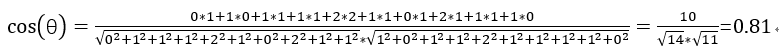根据余弦相似度，句子A和句子B相似度很高。

代码如下：1 import jieba
2 import math
3 s1 = '这只皮靴号码大了。那只号码合适'
4 s1_cut = [i for i in jieba.cut(s1, cut_all=True) if i != '']
5 s2 = '这只皮靴号码不小，那只更合适'
6 s2_cut = [i for i in jieba.cut(s2, cut_all=True) if i != '']
7 print(s1_cut)
8 print(s2_cut)
9 word_set = set(s1_cut).union(set(s2_cut))
10 print(word_set)
11
12 word_dict = dict()
13 i = 0
14 for word in word_set:
15     word_dict[word] = i
16     i += 1
17 print(word_dict)
18
19 s1_cut_code = [word_dict[word] for word in s1_cut]
20 print(s1_cut_code)
21 s1_cut_code = *len(word_dict)
22
23 for word in s1_cut:
24     s1_cut_code[word_dict[word]]+=1
25 print(s1_cut_code)
26
27 s2_cut_code = [word_dict[word] for word in s2_cut]
28 print(s2_cut_code)
29 s2_cut_code = *len(word_dict)
30 for word in s2_cut:
31     s2_cut_code[word_dict[word]]+=1
32 print(s2_cut_code)
33
34 # 计算余弦相似度
35 sum = 0
36 sq1 = 0
37 sq2 = 0
38 for i in range(len(s1_cut_code)):
39     sum += s1_cut_code[i] * s2_cut_code[i]
40     sq1 += pow(s1_cut_code[i], 2)
41     sq2 += pow(s2_cut_code[i], 2)
42
43 try:
44     result = round(float(sum) / (math.sqrt(sq1) * math.sqrt(sq2)), 2)
45 except ZeroDivisionError:
46     result = 0.0
47 print(result)总结，例子很简单，只是比较短短两句话的相似度，算是机器学习入门的一个小案例。可以看到这是使用机器学习算法处理业务的通用步骤，先是取特征，然后特征向量化，最后代入数学公式中。

欢迎关注本人的公众号：
python_ai_bigdata，了解更多关于大数据和机器学习的知识。


展开全文• 基于TF-IDF算法、余弦相似度算法实现相似文本推荐——文本相似度算法，主要应用于文本聚类、相似文本推荐等场景。设计说明使用jieba切词，设置自定义字典使用TF-IDF算法，找出文章的关键词；每篇文章各取出若干个...基于TF-IDF算法、余弦相似度算法实现相似文本推荐——文本相似度算法，主要应用于文本聚类、相似文本推荐等场景。
设计说明
使用jieba切词，设置自定义字典
使用TF-IDF算法，找出文章的关键词；
每篇文章各取出若干个关键词（比如20个），合并成一个集合，计算每篇文章对于这个集合中的词的词频（待优化:为了避免文章长度的差异，可以使用相对词频,）；
生成两篇文章各自的词频向量；
计算两个向量的余弦相似度，值越大就表示越相似。
实现说明
1）初始化
需要对原始文档做些简单的处理，在预处理结果文件中每一行记录一个文档，文档ID与文档内容通过一定分隔符分割（比如以:::间隔），写入txt文档，当然并非一定如此处理，此处可以根据实际使用，调整代码实现。
我选取了五则新闻，其中1,2,3为房地产板块新闻，4,5为NBA板块新闻。原始文本预处理
其余初始化内容详见代码注释。
class DocumentSimilarity:
def __init__(self):
#停词字典路径
self.stopword = 'Cstopword.dic'
#原始文档路径，每一行一个文档，文档ID与文档内容以:::间隔
self.DocumentFilePath = 'Document.txt'
#切词结果存放路径
self.CutWordFilePath = 'CutWordNews.txt'
#每个文档选取关键词个数
self.KeyWordsNum = 10
#推荐相似文档个数
self.DocumentSimilarityNum = 6
#切词结果，格式为{文档ID:切词结果，……}
self.CutWordDict = {}
#文档关键词字典，格式为{文档ID:{关键词：TF-IDF值,……},……}
self.DocumentKeywords = {}
#文档词频字典，格式为{文档ID:{切词：词频,……},……}
self.WordsFrequency = {}
# 导入自定义字典
2）使用jieba切词
之前在
Python 中文分词——jieba
文章中已详细介绍过，这里不做赘述。
def CutWord(self):
"""
切词
:return:
"""
stopwords = [line.strip().decode('utf-8') for line in open(self.stopword).readlines()] # 读取中文停用词表
with open(self.CutWordFilePath, 'w+') as writerfile:
for line in content:
cut_words = jieba.cut(line.split(':::')) #分词,默认是精确分词
tmp = []
for word in cut_words:
word = ''.join(re.findall(u'[\\u4e00-\\u9fa5]|[0-9]+', word)).strip() # 过滤不是中文、数字的内容
if (len(word) != 0 and not stopwords.__contains__(word)): # 去掉在停用词表中出现的内容
tmp.append(word)
writerfile.write('\t'.join(tmp).encode('utf-8') + "\n")
self.CutWordDict[line.split(':::')] = '\t'.join(tmp).encode('utf-8')结巴分词结果
3）使用TF-IDF算法，找出文章的关键词
TF-IDF（term frequency–inverse document frequency）是一种用于信息检索与数据挖掘的常用加权技术。用以评估一字词对于一个文件集或一个语料库中的其中一份文件的重要程度。字、词语的重要性随着它在文件中出现的次数成正比增加，但同时会随着它在语料库中出现的频率成反比下降。即如果某个词或短语在一篇文章中出现的频率（TF）高，并且在整个语料库章中很少出现，则认为此词或者短语具有很好的类别区分能力，适合提取为文档关键字。
计算词频
词频 = 某个词在文章中出现的总次数/文章的总词数
计算逆文档频率
在此，首先需要一个语料库来模拟语言的使用环境。
逆文档频率（IDF） = log（词料库的文档总数/包含该词的文档数+1）
计算TF-IDF值
TF-IDF值 = TF * IDF（TF-IDF值与该词的出现频率成正比，与在整个语料库中的出现次数成反比）
排序取关键字
计算出文章中每个词的TF-IDF值之后，进行排序，选取其中值最高的几个作为关键字。
def GetKeyWords(self):
"""
获取文档关键词
:return:
"""
vectorizer = CountVectorizer() # 将文本中的词语转换为词频矩阵 矩阵元素a[i][j] 表示j词在i类文本下的词频 http://scikit-learn.org/stable/modules/feature_extraction.html
FrequencyMatrix = vectorizer.fit_transform(self.CutWordDict.values()) #返回词频矩阵
transformer = TfidfTransformer()
TFIDF = transformer.fit_transform(FrequencyMatrix) # 第一个fit_transform是计算tf-idf 第二个fit_transform是将文本转为词频矩阵,
AllWord = vectorizer.get_feature_names()
Weight = TFIDF.toarray() # tf-idf矩阵，元素w[i][j]表示j词在i类文本中的tf-idf权重
Title = self.CutWordDict.keys()
self.WordsFrequency = {}
for i in range(len(Weight)): #打印每类文本的tf-idf词语权重，第一个for遍历所有文本，第二个for便利某一类文本下的词语权重
tmp ={}
for j in range(len(AllWord)):
if Weight[i][j] != 0.0:
tmp[AllWord[j]] = Weight[i][j]
sortdict = dict(sorted(tmp.items(), key=lambda d: d, reverse=True)[:self.KeyWordsNum])
self.DocumentKeywords[Title[i]] = sortdict #获取每篇文章的关键词
wordsFrequencyTmp = {}
for word in AllWord:
y = vectorizer.vocabulary_.get(word)
WordFrequency = FrequencyMatrix.toarray()[i][y]
if WordFrequency != 0:
wordsFrequencyTmp[word] = WordFrequency
self.WordsFrequency[Title[i]] = wordsFrequencyTmp4)生成两篇文章各自的词频向量,计算两个向量的余弦相似度，值越大就表示越相似。
@staticmethod
def Cos(cipin):
"""
余弦计算，返回余弦相似度
:param cipin:词频，格式[[1,2],[1,2]]
:return:
"""
dot_product = 0.0
normA = 0.0
normB = 0.0
for x,y in cipin:
dot_product += float(x) * float(y)
normA += float(x) * float(x)
normB += float(y) * float(y)
if normA == 0.0 or normB == 0.0:
return 0
else:
return float(dot_product)/ float(sqrt(normA * normB))
def Cosinesimilarity(self,OneselfTextId):
"""
获取相似文档
:param OneselfTextId:文档ID
:return:
"""
SimilarText ={}
for TextId,Keywords in self.DocumentKeywords.iteritems():
if TextId != OneselfTextId:
Bothtextfrequency = []
K = self.DocumentKeywords[OneselfTextId].keys() + self.DocumentKeywords[TextId].keys()#获取双方关键词列表
for keyword in K :#获取关键词词频
if keyword in self.WordsFrequency[OneselfTextId].keys():
FrequencyOneself =self.WordsFrequency[OneselfTextId][keyword]
else:
FrequencyOneself = 0
if keyword in self.WordsFrequency[TextId].keys():
FrequencyOther =self.WordsFrequency[TextId][keyword]
else:
FrequencyOther = 0
Bothtextfrequency.append([FrequencyOneself,FrequencyOther])
Cosinesimilarity = DocumentSimilarity.Cos(Bothtextfrequency)#计算余弦
SimilarText[TextId] = Cosinesimilarity
SortDict = dict(sorted(SimilarText.items(), key=lambda d: d, reverse=True)[:self.DocumentSimilarityNum])
for i,n in sorted(SimilarText.items(), key=lambda d: d, reverse=True)[:self.DocumentSimilarityNum]:
print u'【文本ID】:{0},【文本相似度】：{1} 【文本关键词】：{2}|{3}'.format(i ,n,','.join(self.DocumentKeywords[i]),','.join(self.DocumentKeywords[OneselfTextId]))
return SortDict使用说明
需安装jieba、sklearn、numpy第三方库。
import re
import jieba
import jieba.analyse
from sklearn.feature_extraction.text import TfidfTransformer
from sklearn.feature_extraction.text import CountVectorizer
from numpy import *
寻找与第一则新闻相似的文本内容结果如下：
if __name__ == "__main__":
DS = DocumentSimilarity()
DS.CutWord()
DS.GetKeyWords()
DS.Cosinesimilarity('1')转载请说明，若对你有帮助，关注支持一下哦。
展开全文• 因工作需要比较两个两个字符串的相似度比较，由于最短编辑距离算法不符合需求，就又找其他算法，在网上看到了另一个算法：余弦相似度算法。于是学习了一下，并写篇博客保存，以便学习以及日后用到。 代码如下: ...
因工作需要比较两个两个字符串的相似度比较，由于最短编辑距离算法不符合需求，就又找其他算法，在网上看到了另一个算法：余弦相似度算法。于是学习了一下，并写篇博客保存，以便学习以及日后用到。 代码如下:
import java.util.HashMap
import java.util.Map;
import java.util.Set;

/*
* 计算两个字符串(英文字符)的相似度，简单的余弦计算，未添权重
*/

public class AnotherCompare {
Map<Character, int[]> vectorMap = new HashMap<Character, int[]>();

int[] tempArray = null;

public AnotherCompare(String string1, String string2) {

for (Character character1 : string1.toCharArray()) {  //拆解为向量
if (vectorMap.containsKey(character1)) {   //该字符是否已经存在map中
vectorMap.get(character1)++;   //key对应的值是一个数组，第一个字符串的字符出现次数存储在数组第一个位置
} else {
tempArray = new int;
tempArray = 1;  //如果字符没有出现过，就添加到map中，数组第一个位置设为1
tempArray = 0;
vectorMap.put(character1, tempArray);
}
}
for (Character character2 : string2.toCharArray()) {  //拆解为向量
if (vectorMap.containsKey(character2)) {
vectorMap.get(character2)++;
} else {
tempArray = new int;
tempArray = 0;
tempArray = 1;  //第二个字符串的字符出现次数存储在数组的第二个位置
vectorMap.put(character2, tempArray);
}
}
/*
* 到最后，map里的key对应的数组，第一个位置存储的是第一个字符串字符对应的向量，第二个位置存储的是第二个字符串字符对应的向量。
*/
}

// 求余弦相似度
public double sim() {   //分子分母相除
double result = 0;
result = pointMulti(vectorMap) / sqrtMulti(vectorMap);
return result;    //余弦度结果返回,因为是余弦，返回结果越大，夹角越小，两个向量方向越接近，即两个字符串越相似
}

//求平方根
private double sqrtMulti(Map<Character, int[]> paramMap) {
double result = 0;
result = squares(paramMap);  //先求平方和
result = Math.sqrt(result);  //再开根号，就是求模
return result;
}

// 求平方和，分母上，向量求模的平方
private double squares(Map<Character, int[]> paramMap) {
double result1 = 0;
double result2 = 0;
Set<Character> keySet = paramMap.keySet();
for (Character character : keySet) {
int temp[] = paramMap.get(character);  //获取key对应的值--数组
result1 += (temp * temp);   //temp存储的是第一个字符串对应的向量
result2 += (temp * temp);   //temp存储的是第二个字符串对应的向量
}
return result1 * result2;
}

// 点乘法，在分子上，向量相乘
private double pointMulti(Map<Character, int[]> paramMap) {
double result = 0;
Set<Character> keySet = paramMap.keySet();  //返回map中所有key值的列表，这里的set，也可以用list代替吧
for (Character character : keySet) {    //存储的key值都是不重复的
int temp[] = paramMap.get(character);   //获取key对应的值
result += (temp * temp);
}
return resul

}

原理网上都有，这个代码也是网上的代码，我只是学习，加上了自己理解的注释。如有不足，请多指教。
展开全文java
• 在工作中一直使用余弦相似度算法计算两段文本的相似度和两个用户的相似度。一直弄不明白多维的余弦相似度公式是怎么推导来的。今天终于花费时间把公式推导出来，其实很简单，都是高中学过的知识，只是很多年没用了，...
• 衡量两个向量x、y的相关性(夹角的余弦) MATLAB 代码余弦相似度也被⼀些迁移学习研究所使用。
• 算法是用于文本相似的判定，同时也可以判定两个用户的相似性。算法是以C#实现的，封装完毕，如有急要可以留言哦
• 在推荐系统中，对于文章内容和物品相似度有多种算法可以使用，而余弦相似度算法相对简单并且准确性也不错，因此使用率比较高。 余弦相似度用向量空间中两个向量夹角的余弦值作为衡量两个个体间差异的大小。余弦值越...
• 文档所给的是余弦相似度计算代码，在文本分类中用来计算相似度值，以此对文本分类
• // 求余弦相似度 public double sim() { double result = 0; result = pointMulti(vector1, vector2) / sqrtMulti(vector1, vector2); return result; } public static double simd() { double result...
• 1、余弦相似度 余弦距离，也称为余弦相似度，是用向量空间中两个向量夹角的余弦值作为衡量两个个体间差异的大小的度量。余弦值越接近1，就表明夹角越接近0度，也就是两个向量越相似，这就叫"余弦相似性"。 上...
• 文章目录自然语言处理系列三十文本相似度算法余弦相似度Java代码实现总结 自然语言处理系列三十 文本相似度算法 在自然语言处理中，我们经常需要判定两个东西是否相似。比如，在微博的热点话题推荐那里，我们需要...人工智能 java python 编程语言
• 做密码相似度计算是一个抽象的过程，传统的java代码无法处理这种抽象的计算。只有通过数学建模，建立一个比较两对象的相似度模型，把比较的两者密码换算成两个向量，把历史密码中的出现的字符作为向量...java
• 文章目录自然语言处理系列三十一文本相似度算法余弦相似度Python代码实现总结 自然语言处理系列三十一 文本相似度算法 在自然语言处理中，我们经常需要判定两个东西是否相似。比如，在微博的热点话题推荐那里，我们...人工智能 字符串 python java
• ## 相似度算法之余弦相似度

万次阅读 多人点赞 2016-07-16 20:50:04
余弦距离，也称为余弦相似度，是用向量空间中两个向量夹角的余弦值作为衡量两个个体间差异的大小的度量。 余弦值越接近1，就表明夹角越接近0度，也就是两个向量越相似，这就叫"余弦相似性"。 上图两个向量a,b的...
• 文章目录自然语言处理系列二十九文本相似度算法余弦相似度算法原理总结 自然语言处理系列二十九 文本相似度算法 在自然语言处理中，我们经常需要判定两个东西是否相似。比如，在微博的热点话题推荐那里，我们需要...人工智能 大数据 编程语言 python
• 余弦距离，也称为余弦相似度，是用向量空间中两个向量夹角的余弦值作为衡量两个个体间差异的大小的度量。 余弦值越接近1，就表明夹角越接近0度，也就是两个向量越相似，这就叫"余弦相似性"。  上图两...
• n_row = size(A,1); norm_r = sqrt(sum(abs(A).^2,2)); % same as norm(A,2,'rows') B = zeros(n_row,n_row); for i = 1:n_row for j = i:n_row ...如果大家有了解改进的余弦相似度的，欢迎交流哈~ ...
• 文章目录余弦相似度概述一、实现思路二、实现流程三、代码实现总结 概述      用向量空间中两个向量夹角的余弦值作为衡量两个个体间差异的大小的度量。余弦值越接近1，就表明夹角越接近0...
• 基于Lucene3.5版本、TF-IDF、余弦相似实现的文本相似度算法。 详细介绍《》 样本库提取 使用webmagic爬取华为应用市场应用的描述信息，当做样本。 在工程的conf/doc目录有1000多个应用样本。 具体代码实现在工程下面...Java
• 余弦相似度简介 余弦相似度，又称为余弦相似性，是通过计算两个向量的夹角余弦值来评估他们的相似度。对于两个向量，可以想象成空间中的两条线段，都是从原点（[0, 0, ...]）出发，指向不同的方向。两条线段之间...
• 修正余弦相似度，指中心化（减去平均值）后再求余弦相似度。1. 从数学角度看，给定向量X和Y，Pearson相关系数和修正余弦相似度的计算是相同的，它们的关系参考下面的链接: ...协同过滤
• 余弦相似度算法写的c++程序，计算如“我 爱 看 电视”和“我 不 爱 看 电影”之间的余弦相似度。由于没有分词程序，所以需要用空格手动分词...